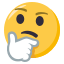## Playing with mathematical conventions and axioms

Use this forum to discuss the philosophy of science. Philosophy of science deals with the assumptions, foundations, and implications of science.
SubatomicAl1en
New Trial Member
Posts: 15
Joined: January 6th, 2020, 9:08 am

### Playing with mathematical conventions and axioms

Let's say that I am a time traveller, and I travel all the way back to when civilization started. I start teaching everyone 1 = 2, so everyone starts developing math theories that are based on the assumption of 1 = 2. Will the answers still be "right"? Will every mathematical equation like Maxwells equation and Schrodingers equation just be written in a different way:? Or will there be actual different equations and laws of nature?
What I'm saying is, is there a direct correspondence between nature and our conventions and assumed axioms?

h_k_s
Posts: 1138
Joined: November 25th, 2018, 12:09 pm
Favorite Philosopher: Aristotle
Location: Rocky Mountains

### Re: Playing with mathematical conventions and axioms

SubatomicAl1en wrote:
August 1st, 2020, 12:15 am
Let's say that I am a time traveller, and I travel all the way back to when civilization started. I start teaching everyone 1 = 2, so everyone starts developing math theories that are based on the assumption of 1 = 2. Will the answers still be "right"? Will every mathematical equation like Maxwells equation and Schrodingers equation just be written in a different way:? Or will there be actual different equations and laws of nature?
What I'm saying is, is there a direct correspondence between nature and our conventions and assumed axioms?
First, there is no such real thing as "time travel." It is a figment of pulp fiction in science.

Secondly, mathematics does not exist either. It is merely a game people play in their minds with definitions.

Mathematics probably began during the stone age when hunters were counting game animals in a herd, or when gatherers were counting seeds, nuts, and fruits gathered for the tribe or clan.

Mathematics probably began with human fingers associated with counting, such as I, II, III, IIII, IIIII, etc.

Then someone probably made shortcuts such as I, II, III, IV, V, VI, VII, VIII, IX, X, etc. We know from history that the Egyptians and Greeks used a base 10, which probably came from their 10 fingers or 10 toes, and that the Babylonians use a base 7 from the 5 visible planets in the night sky together with Sun and Moon.

You can use any base you want.

Thus in your primitive example, 1 cannot ever equal 2. That would be a contradiction. And in philosophy whenever you assume something in an attempt to prove it, if you arrive at a contradiction, then the assumption is determined to be false.

Do NOT confuse science fiction with philosophy.

Q.E.D.

Pattern-chaser
Posts: 875
Joined: September 22nd, 2019, 5:17 am
Favorite Philosopher: Heidegger
Location: England

### Re: Playing with mathematical conventions and axioms

SubatomicAl1en wrote:
August 1st, 2020, 12:15 am
Let's say that I am a time traveller, and I travel all the way back to when civilization started. I start teaching everyone 1 = 2, so everyone starts developing math theories that are based on the assumption of 1 = 2. Will the answers still be "right"? Will every mathematical equation like Maxwells equation and Schrodingers equation just be written in a different way:? Or will there be actual different equations and laws of nature?
1 + 1 = 2 is true only because we define it as such, provided we accept the axioms (i.e. assumptions) of number and set theory. If you want to teach that "1 = 2", you will also need to define your new number theory, to describe how it makes sense, and is consistent, and maybe even logical.Oh, and how it is useful.SubatomicAl1en wrote:
August 1st, 2020, 12:15 am
What I'm saying is, is there a direct correspondence between nature and our conventions and assumed axioms?
Yes. Nature is the Master, the Reference, and our conventions and axioms are our attempts to describe it usefully. This is the only correspondence that exists. You seem to be wondering if we can change the universe by changing our description(s) of it....Pattern-chaser

"Who cares, wins"